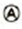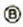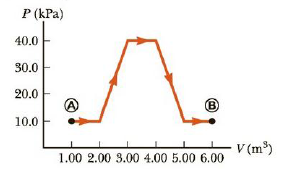Chapter 12, Problem 71AP

Chapter
Section
Textbook Problem

A cylinder containing 10.0 moles of a monatomic ideal gas expands fromtoalong the path shown in Figure P12.71. (a) Find the temperature of the gas at point A and the temperature at point. (b) How much work is done by the gas during this expansion? (c) What is the change in internal energy of the gas? (d) Find the energy transferred to the gas by heat in this process.Figure P12.71

(a)

To determine
the temperature of the gas at point A and point B

Explanation

Section1:

To determine: the temperature of the gas at point A.

Answer: The temperature of the gas at point A is 1.20×102K .

Explanation:

Given Info:

The amount of ideal gas is 10mol .

Pressure of gas at the point A is 10KPa .

Volume of the gas at the point A is 1.0m3 .

Formula to calculate the temperature is,

TA=PAVAnR

• PA is the pressure of the gas at point A
• VA is the volume of gas at point A
• n is the amount of gas in moles
• R is universal gas constant

Substitute 10KPa for PA , 1.0m3 for VA , 10mol for n and 8.31J/molK for R to find the temperature of the gas at point A,

TA=(10.0×103Pa)(1.00m3)(10.0mol)(8.31J/molK)=1.20×102K

Thus, the temperature of the gas at point A is 1.20×102K .

Section 2:

To determine: The temperature of the gas at point B

(b)

To determine
The work done during the expansion.

(c)

To determine
The change in internal energy of the gas.

(d)

To determine
The energy transferred to the gas by heat.

Still sussing out bartleby?

Check out a sample textbook solution.

See a sample solution

The Solution to Your Study Problems

Bartleby provides explanations to thousands of textbook problems written by our experts, many with advanced degrees!

Get Started

Adolescents are likely to grow out of early disordered eating behaviors by young adulthood. T F

Nutrition: Concepts and Controversies - Standalone book (MindTap Course List)

What is the maximum number of bonds that a carbon atom can form?

Biology: The Dynamic Science (MindTap Course List)

True or false? Action potentials vary in their size

Biology: The Unity and Diversity of Life (MindTap Course List)